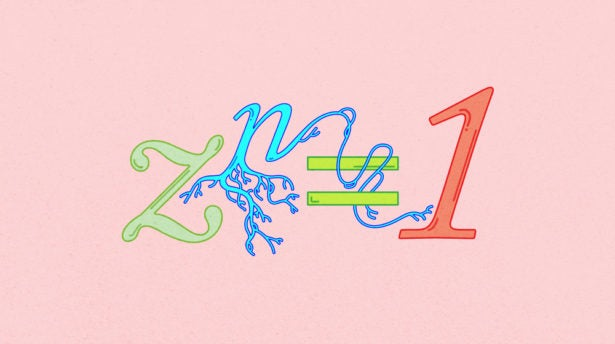# The Almost-Proof of Fermat’s Last Theorem

19th-century mathematicians thought the “roots of unity” were the key to solving Fermat’s Last Theorem. Then they discovered a fatal flaw.Explore

Sometimes the usual numbers aren’t enough to solve a problem. Mathematicians in the 19th century understood this when they tried to crack Fermat’s Last Theorem, which by then had been around for 200 years and was considered the biggest open problem in number theory.

Fermat’s Last Theorem predicts that there are no positive integer solutions to equations of the form abcn for n greater than 2. It seemed impossible to prove this statement by working with just the regular whole numbers (1, 2, 3 … ), so mathematicians went searching for more exotic values they could incorporate among the standard ones to improve the situation.

They found them. In fact, they thought that to prove Fermat’s Last Theorem, they needed to create a number system that included a wild-looking value: ei/n. It’s a mashup of famous math terms including the natural logarithm e, π, and the imaginary number i, yet despite that outward complexity, it reduces to a very simple idea. The value, when you sort through all the pieces, is equivalent to what’s called the nth root of unity—a number that you multiply with itself n times to get 1.

When you’re working with integers, there are only two roots of one: 1 and ‑1. But when you’re working with complex numbers (numbers that include a real part and an imaginary part), there are many. And those values—all the roots of unity—seemed to unlock Fermat’s Last Theorem. By mixing them in with the standard numbers, mathematicians were able to break the equations described by Fermat into simpler pieces and prove that there are no whole numbers greater than 2 that satisfy them.

Except there was a catch. As my story “New Number Systems Seek Their Lost Primes” describes, by expanding the number system to include new values, mathematicians lost something essential: unique prime factorization. Primes are the atoms of a number system — its fundamental building blocks — and unique prime factorization ensures that any number, such as 12, can be expressed uniquely as a product of primes: 2 x 2 x 3. The expanded number systems used to solve Fermat’s Last Theorem yielded competing prime factorizations, making these systems an ultimately shaky basis on which to construct a proof.

“Even today, in many false proofs of Fermat’s Last Theorem found by amateurs, somewhere or other this is the mistake — they’re assuming in some of these bigger number systems that numbers can be uniquely decomposed into primes,” said Manjul Bhargava, a mathematician at Princeton University. “It’s so counterintuitive to think that could fail for a bigger number system, but it sometimes does.”